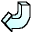Back### Solution 2

3x + 2 = 11

Subtract 2 from both sides

3x + 2 - 2 = 11 - 2

3x = 9

Divide both sides by 3

x = 3

3 x 3 + 2 = 11